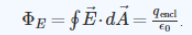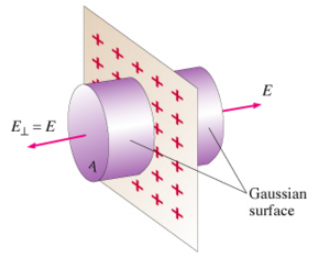# Problem: Gauss's law relates the electric flux ΦE through a closed surface to the total charge qencl enclosed by the surface:You can use Gauss's law to determine the charge enclosed inside a closed surface on which the electric field is known. However, Gauss's law is most frequently used to determine the electric field from a symmetric charge distribution.Now consider the case with one effective direction. In order to make a problem effectively one-dimensional, it is necessary to extend a charge to infinity along two orthogonal axes, conventionally taken to be x and y. When the change is extended to infinity in the xy plane (so that by symmetry, the electric field will be directed in the z-direction and depend only on z), the charge distribution is sometimes a sheet charge. The symbol usually used for two-dimensional charge density is either δ or η. In this problem we will use δ, δ has units of coulombs per meter squared.In solving the magnitude of the electric field E(z) produced by a sheet charge with a charge density δ, use the planar symmetry since the charge distribution doesn't change if you slide it in any direction of xy plane parallel to the sheet. Therefore, at each point, the electric field is perpendicular to the sheet and must have the same magnitude at any given distance on either side of the sheet. To take advantage of these symmetry properties, use a Gaussian surface in the shape of a cylinder with its axis perpendicular to the sheet of charge, with ends of area A which will cancel out of the expression E(z) in the end. The result of applying Gauss's law to this situation then gives an expression for E(z) for both z &gt; 0 and z &lt; 0.Express E(z) for z &gt; 0 in terms of some or all of the variable/constants δ, z, and ε0.

###### FREE Expert Solution

In this case, E(z) = E(2A)

90% (429 ratings)###### Problem Details

Gauss's law relates the electric flux ΦE through a closed surface to the total charge qencl enclosed by the surface:You can use Gauss's law to determine the charge enclosed inside a closed surface on which the electric field is known. However, Gauss's law is most frequently used to determine the electric field from a symmetric charge distribution.

Now consider the case with one effective direction. In order to make a problem effectively one-dimensional, it is necessary to extend a charge to infinity along two orthogonal axes, conventionally taken to be x and y. When the change is extended to infinity in the xy plane (so that by symmetry, the electric field will be directed in the z-direction and depend only on z), the charge distribution is sometimes a sheet charge. The symbol usually used for two-dimensional charge density is either δ or η. In this problem we will use δ, δ has units of coulombs per meter squared.

In solving the magnitude of the electric field E(z) produced by a sheet charge with a charge density δ, use the planar symmetry since the charge distribution doesn't change if you slide it in any direction of xy plane parallel to the sheet. Therefore, at each point, the electric field is perpendicular to the sheet and must have the same magnitude at any given distance on either side of the sheet. To take advantage of these symmetry properties, use a Gaussian surface in the shape of a cylinder with its axis perpendicular to the sheet of charge, with ends of area A which will cancel out of the expression E(z) in the end. The result of applying Gauss's law to this situation then gives an expression for E(z) for both z > 0 and z < 0.Express E(z) for z > 0 in terms of some or all of the variable/constants δ, z, and ε0.